SSC BOARD PAPERS IMPORTANT TOPICS COVERED FOR BOARD EXAM 2024

### Practice Set 5.1 Chapter 5 - Quadrilaterals Mathematics Part II Solutions for Class 9 Math

##### Class 9th Mathematics Part Ii MHB Solution
###### Practice Set 5.1
Question 1.

Diagonals of a parallelogram WXYZ intersect each other at point O. If ∠ XYZ = 135° then what is the measure of ∠XWZ and ∠YZW? If l(OY)= 5 cm then l(WY)=?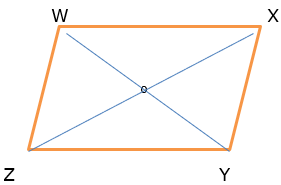Given ZX and WY are the diagonals of the parallelogram

∠ XYZ = 135° ⇒ ∠ XWZ = 135° as the opposite angels of a parallelogram are congruent.

∠YZW + ∠ XWZ = 180° as the adjacent angels of the parallelogram are supplementary.

⇒ ∠YZW = 180° - 135° = 45°

Length of OY = 5 cm then length of WY = WO + OY = 5+5 = 10 cm

(diagonals of the parallelogram bisect each other. So, O is midpoint of WY)

Question 2.

In a parallelogram ABCD, If ∠A = (3x +12)°, ∠B = (2x -32) ° then find the value of x and then find the measures of ∠C and ∠D.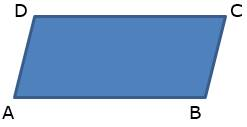∠A = (3x +12)°

∠B = (2x -32) °

∠A + ∠B = 180° (supplementary angles of the ∥gram)

(3x +12) + (2x -32) = 180

5x – 20 = 180°

5x = 200°

∴ x= 40°

∠A = (3 × 40) +12 = 120 + 12

= 132

⇒ ∠C = 132° (opposite ∠s are congruent)

Similarly, ∠B = 2× 40 – 32

= 80 - 32°

= 48°

⇒ ∠D = 48°(opposite ∠s are congruent)

Question 3.

Perimeter of a parallelogram is 150 cm. One of its sides is greater than the other side by 25 cm. Find the lengths of all sides.

perimeter of parallelogram = 150cm

Let the one side of parallelogram be x cm then

Acc. To the given condition

Other side is (x+25) cm

Perimeter of parallelogram = 2(a+b)

150 = 2( x+x+25)

150 = 2(2x+25)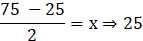One side is 25cm and the other side is 50cm.

Question 4.

If the ratio of measures of two adjacent angles of a parallelogram is 1 : 2, find the measures of all angles of the parallelogram.

Given that the ratio of measures of two adjacent angles of a parallelogram= 1 : 2

If one ∠ is x other would be 180 – x as the adjacent ∠s of a parallelogram are supplementary.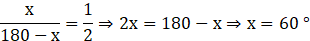Other ∠ is 120° .

The measure of all the angles are 60 °, 120 °, 60 ° and 120 ° where 60 ° and 120 ° are adjacent ∠s and 60 ° and 60 ° are congruent opposite angles.

Question 5.

Diagonals of a parallelogram intersect each other at point O. If AO = 5, BO = 12 and AB = 13 then show that 􀀍ABCD is a rhombus.

The figure is given below: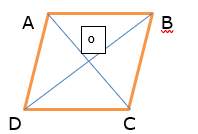Given AO =5, BO = 12 and AB = 13

In Δ AOB, AO2 + BO2 = AB2

∵ 52 + 122 = 132

252 + 1442 = 1692

so by the Pythagoras theorem

Δ AOB is right angled at ∠ AOB.

But ∠ AOB + ∠ AOD forms a linear pair so the given parallelogram is rhombus whose diagonal bisects each other at 90°.

Question 6.

In the figure 5.12, 􀀍PQRS and 􀀍ABCR are two parallelograms. If ∠P = 110° then find the measures of all angles of 􀀍ABCR.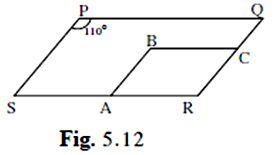given PQRS and ABCR are two ∥gram.

∠P = 110° ⇒ ∠R = 110°

(opposite ∠s of parallelogram are congruent)

Now if , ∠R = 110° ⇒ ∠ B = 110°

∠B + ∠A = 180°

(adjacent ∠s of a parallelogram are supplementary)

⇒ ∠A = 70° ⇒ ∠C = 70°

(opposite ∠s of parallelogram are congruent)

Question 7.

In figure 5.13 􀀍ABCD is a parallelogram. Point E is on the ray AB such that BE = AB then prove that line ED bisects seg BC at point F.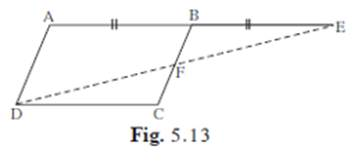Given, 􀀍ABCD is a parallelogram

And BE = AB

But AB = DC (opposite sides of the parallelogram are equal and parallel)

⇒ DC = BE

In Δ BEF and ∠DCF

∠DFC = ∠BFE (vertically opposite angles)

∠DFC = ∠ BFE (alternate ∠s on the transversal BC with AB and DC as ∥ )

And BE = AB (given)

Δ BEF ≅ ∠DCF (by AAS criterion)

⇒ BF =FC (corresponding parts of the congruent triangles)

⇒ F is mid-point of the line BC. Hence proved.